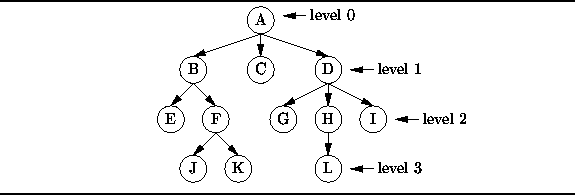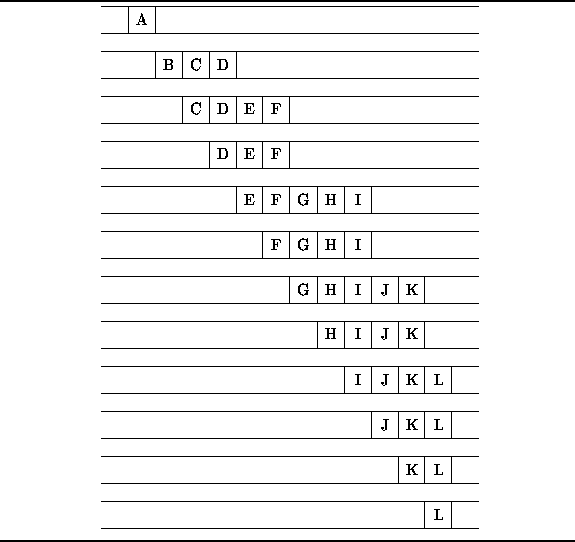Data Structures and Algorithms with Object-Oriented Design Patterns in C### Applications

The FIFO nature of queues makes them useful in certain algorithms. For example, we will see in Chapterthat a queue is an essential data structure for many different graph algorithms. In this section we illustrate the use of a queue in the breadth-first traversal  of a tree.

Figureshows an example of a tree. A tree is comprised of nodes  (indicated by the circles) and edges  (shown as arrows between nodes). We say that the edges point from the parent  node to a child  node. The degree  of a node is equal to the number of children of that node. For example, node A in Figurehas degree three and its children are nodes B, C, and D. A child and all of its descendents is called a subtree .Figure: A tree.

One way to represent such a tree is to use a collection of linked structures. Consider the following interface definition which is an abridged version of the Tree interface described in Chapter.

```public interface Tree
{
object Key { get; }
int Degree { get; }
Tree GetSubtree(int i);
// ...
};```

Each node in a tree is represented by an object that implements the Tree interface. The Key property provides a get accessor that returns an object which represents the contents of the node. E.g. in Figure, each node carries a one-character label so the Key property would return a char value that represents that label. The Degree property provides a get accessor that returns the degree of the node and the GetSubtree method takes an int argument i and returns the corresponding child of that node.

One of the essential operations on a tree is a tree traversal . A traversal visits one-by-one all the nodes in a given tree. To visit a node means to perform some computation using the information contained in that node--e.g., print the key. The standard tree traversals are discussed in Chapter. In this section we consider a traversal which is based on the levels of the nodes in the tree.

Each node in a tree has an associated level which arises from the position of that node in the tree. For example, node A in Figureis at level 0, nodes B, C, and D are at level 1, etc. A breadth-first traversal  visits the nodes of a tree in the order of their levels. At each level, the nodes are visited from left to right. For this reason, it is sometimes also called a level-order traversal . The breadth-first traversal of the tree in Figurevisits the nodes from A to L in alphabetical order.

One way to implement a breadth-first traversal of a tree is to make use of a queue as follows: To begin the traversal, the root node of the tree is enqueued. Then, we repeat the following steps until the queue is empty:

1. Dequeue and visit the first node in the queue.
2. Enqueue its children in order from left to right.
Figureillustrates the breadth-first traversal algorithm by showing the contents of the queue immediately prior to each iteration.Figure: Queue contents during the breadth-first traversal of the tree in Figure.Copyright © 2001 by Bruno R. Preiss, P.Eng. All rights reserved.